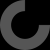MakerBot Print is our newest print-prepration software, which supports native CAD files and STL assemblies,
allows you to interact with all your printers via the Cloud, and many other exciting new features.

Download Now

# Report as inappropriate

Failed to authenticate application: 404: "<!DOCTYPE html PUBLIC "-//W3C//DTD HTML 4.01//EN" "http://www.w3.org/TR/html4/strict.dtd">\n\n\n\n\t404\n\t\n//<![CDATA[\nwindow.__mirage2 = {petok:"491f277db60dd8a1e294379f3d95a28170730a53-1513796297-1800"};\n//]]>\n\n\n(window.NREUM||(NREUM={})).loader_config={xpid:"UQMGU1NACwsIXFBQ"};window.NREUM||(NREUM={}),__nr_require=function(t,n,e){function r(e){if(!n[e]){var o=n[e]={exports:{}};t[e].call(o.exports,function(n){var o=t[e][n];return r(o||n)},o,o.exports)}return n[e].exports}if("function"==typeof __nr_require)return __nr_require;for(var o=0;o0&&(p-=1)}),s.on("internal-error",function(t){i("ierr",[t,c.now(),!0])})},{}],3:[function(t,n,e){t("loader").features.ins=!0},{}],4:[function(t,n,e){function r(t){}if(window.performance&&window.performance.timing&&window.performance.getEntriesByType){var o=t("ee"),i=t("handle"),a=t(8),s=t(7),c="learResourceTimings",f="addEventListener",u="resourcetimingbufferfull",d="bstResource",l="resource",p="-start",h="-end",m="fn"+p,w="fn"+h,v="bstTimer",y="pushState",g=t("loader");g.features.stn=!0,t(6);var b=NREUM.o.EV;o.on(m,function(t,n){var e=t;e instanceof b&&(this.bstStart=g.now())}),o.on(w,function(t,n){var e=t;e instanceof b&&i("bst",[e,n,this.bstStart,g.now()])}),a.on(m,function(t,n,e){this.bstStart=g.now(),this.bstType=e}),a.on(w,function(t,n){i(v,[n,this.bstStart,g.now(),this.bstType])}),s.on(m,function(){this.bstStart=g.now()}),s.on(w,function(t,n){i(v,[n,this.bstStart,g.now(),"requestAnimationFrame"])}),o.on(y+p,function(t){this.time=g.now(),this.startPath=location.pathname+location.hash}),o.on(y+h,function(t){i("bstHist",[location.pathname+location.hash,this.startPath,this.time])}),f in window.performance&&(window.performance["c"+c]?window.performance[f](u,function(t){i(d,[window.performance.getEntriesByType(l)]),window.performance["c"+c]()},!1):window.performance[f]("webkit"+u,function(t){i(d,[window.performance.getEntriesByType(l)]),window.performance["webkitC"+c]()},!1)),document[f]("scroll",r,{passive:!0}),document[f]("keypress",r,!1),document[f]("click",r,!1)}},{}],5:[function(t,n,e){function r(t){for(var n=t;n&&!n.hasOwnProperty(u);)n=Object.getPrototypeOf(n);n&&o(n)}function o(t){s.inPlace(t,[u,d],"-",i)}function i(t,n){return t}var a=t("ee").get("events"),s=t(18)(a,!0),c=t("gos"),f=XMLHttpRequest,u="addEventListener",d="removeEventListener";n.exports=a,"getPrototypeOf"in Object?(r(document),r(window),r(f.prototype)):f.prototype.hasOwnProperty(u)&&(o(window),o(f.prototype)),a.on(u+"-start",function(t,n){var e=t,r=c(e,"nr@wrapped",function(){function t(){if("function"==typeof e.handleEvent)return e.handleEvent.apply(e,arguments)}var n={object:t,"function":e}[typeof e];return n?s(n,"fn-",null,n.name||"anonymous"):e});this.wrapped=t=r}),a.on(d+"-start",function(t){t=this.wrapped||t})},{}],6:[function(t,n,e){var r=t("ee").get("history"),o=t(18)(r);n.exports=r,o.inPlace(window.history,["pushState","replaceState"],"-")},{}],7:[function(t,n,e){var r=t("ee").get("raf"),o=t(18)(r),i="equestAnimationFrame";n.exports=r,o.inPlace(window,["r"+i,"mozR"+i,"webkitR"+i,"msR"+i],"raf-"),r.on("raf-start",function(t){t=o(t,"fn-")})},{}],8:[function(t,n,e){function r(t,n,e){t=a(t,"fn-",null,e)}function o(t,n,e){this.method=e,this.timerDuration=isNaN(t)?0:+t,t=a(t,"fn-",this,e)}var i=t("ee").get("timer"),a=t(18)(i),s="setTimeout",c="setInterval",f="clearTimeout",u="-start",d="-";n.exports=i,a.inPlace(window,[s,"setImmediate"],s+d),a.inPlace(window,[c],c+d),a.inPlace(window,[f,"clearImmediate"],f+d),i.on(c+u,r),i.on(s+u,o)},{}],9:[function(t,n,e){function r(t,n){d.inPlace(n,["onreadystatechange"],"fn-",s)}function o(){var t=this,n=u.context(t);t.readyState>3&&!n.resolved&&(n.resolved=!0,u.emit("xhr-resolved",[],t)),d.inPlace(t,y,"fn-",s)}function i(t){g.push(t),h&&(x?x.then(a):w?w(a):(E=-E,O.data=E))}function a(){for(var t=0;t34||p<10)||window.opera||t.addEventListener("progress",function(t){n.lastSize=t.loaded},!1)}),f.on("open-xhr-start",function(t){this.params={method:t},i(this,t),this.metrics={}}),f.on("open-xhr-end",function(t,n){"loader_config"in NREUM&&"xpid"in NREUM.loader_config&&this.sameOrigin&&n.setRequestHeader("X-NewRelic-ID",NREUM.loader_config.xpid)}),f.on("send-xhr-start",function(t,n){var e=this.metrics,r=t,o=this;if(e&&r){var i=h(r);i&&(e.txSize=i)}this.startTime=a.now(),this.listener=function(t){try{"abort"===t.type&&(o.params.aborted=!0),("load"!==t.type||o.called===o.totalCbs&&(o.onloadCalled||"function"!=typeof n.onload))&&o.end(n)}catch(e){try{f.emit("internal-error",[e])}catch(r){}}};for(var s=0;s<d;s++)n.addEventListener(u[s],this.listener,!1)}),f.on("xhr-cb-time",function(t,n,e){this.cbTime+=t,n?this.onloadCalled=!0:this.called+=1,this.called!==this.totalCbs||!this.onloadCalled&&"function"==typeof e.onload||this.end(e)}),f.on("xhr-load-added",function(t,n){var e=""+l(t)+!!n;this.xhrGuids&&!this.xhrGuids[e]&&(this.xhrGuids[e]=!0,this.totalCbs+=1)}),f.on("xhr-load-removed",function(t,n){var e=""+l(t)+!!n;this.xhrGuids&&this.xhrGuids[e]&&(delete this.xhrGuids[e],this.totalCbs-=1)}),f.on("addEventListener-end",function(t,n){n instanceof m&&"load"===t&&f.emit("xhr-load-added",[t,t],n)}),f.on("removeEventListener-end",function(t,n){n instanceof m&&"load"===t&&f.emit("xhr-load-removed",[t,t],n)}),f.on("fn-start",function(t,n,e){n instanceof m&&("onload"===e&&(this.onload=!0),("load"===(t&&t.type)||this.onload)&&(this.xhrCbStart=a.now()))}),f.on("fn-end",function(t,n){this.xhrCbStart&&f.emit("xhr-cb-time",[a.now()-this.xhrCbStart,this.onload,n],n)})}},{}],11:[function(t,n,e){n.exports=function(t){var n=document.createElement("a"),e=window.location,r={};n.href=t,r.port=n.port;var o=n.href.split("://");!r.port&&o&&(r.port=o.split("/").split("@").pop().split(":")),r.port&&"0"!==r.port||(r.port="https"===o?"443":"80"),r.hostname=n.hostname||e.hostname,r.pathname=n.pathname,r.protocol=o,"/"!==r.pathname.charAt(0)&&(r.pathname="/"+r.pathname);var i=!n.protocol||":"===n.protocol||n.protocol===e.protocol,a=n.hostname===document.domain&&n.port===e.port;return r.sameOrigin=i&&(!n.hostname||a),r}},{}],12:[function(t,n,e){function r(){}function o(t,n,e){return function(){return i(t,[f.now()].concat(s(arguments)),n?null:this,e),n?void 0:this}}var i=t("handle"),a=t(15),s=t(16),c=t("ee").get("tracer"),f=t("loader"),u=NREUM;"undefined"==typeof window.newrelic&&(newrelic=u);var d=["setPageViewName","setCustomAttribute","setErrorHandler","finished","addToTrace","inlineHit","addRelease"],l="api-",p=l+"ixn-";a(d,function(t,n){u[n]=o(l+n,!0,"api")}),u.addPageAction=o(l+"addPageAction",!0),u.setCurrentRouteName=o(l+"routeName",!0),n.exports=newrelic,u.interaction=function(){return(new r).get()};var h=r.prototype={createTracer:function(t,n){var e={},r=this,o="function"==typeof n;return i(p+"tracer",[f.now(),t,e],r),function(){if(c.emit((o?"":"no-")+"fn-start",[f.now(),r,o],e),o)try{return n.apply(this,arguments)}catch(t){throw c.emit("fn-err",[arguments,this,t],e),t}finally{c.emit("fn-end",[f.now()],e)}}}};a("setName,setAttribute,save,ignore,onEnd,getContext,end,get".split(","),function(t,n){h[n]=o(p+n)}),newrelic.noticeError=function(t){"string"==typeof t&&(t=new Error(t)),i("err",[t,f.now()])}},{}],13:[function(t,n,e){n.exports=function(t){if("string"==typeof t&&t.length)return t.length;if("object"==typeof t){if("undefined"!=typeof ArrayBuffer&&t instanceof ArrayBuffer&&t.byteLength)return t.byteLength;if("undefined"!=typeof Blob&&t instanceof Blob&&t.size)return t.size;if(!("undefined"!=typeof FormData&&t instanceof FormData))try{return JSON.stringify(t).length}catch(n){return}}}},{}],14:[function(t,n,e){var r=0,o=navigator.userAgent.match(/Firefox[\/\s](\d+\.\d+)/);o&&(r=+o),n.exports=r},{}],15:[function(t,n,e){function r(t,n){var e=[],r="",i=0;for(r in t)o.call(t,r)&&(e[i]=n(r,t[r]),i+=1);return e}var o=Object.prototype.hasOwnProperty;n.exports=r},{}],16:[function(t,n,e){function r(t,n,e){n||(n=0),"undefined"==typeof e&&(e=t?t.length:0);for(var r=-1,o=e-n||0,i=Array(o<0?0:o);++r<o;)i[r]=t[n+r];return i}n.exports=r},{}],17:[function(t,n,e){n.exports={exists:"undefined"!=typeof window.performance&&window.performance.timing&&"undefined"!=typeof window.performance.timing.navigationStart}},{}],18:[function(t,n,e){function r(t){return!(t&&t instanceof Function&&t.apply&&!t[a])}var o=t("ee"),i=t(16),a="nr@original",s=Object.prototype.hasOwnProperty,c=!1;n.exports=function(t,n){function e(t,n,e,o){function nrWrapper(){var r,a,s,c;try{a=this,r=i(arguments),s="function"==typeof e?e(r,a):e||{}}catch(f){l([f,"",[r,a,o],s])}u(n+"start",[r,a,o],s);try{return c=t.apply(a,r)}catch(d){throw u(n+"err",[r,a,d],s),d}finally{u(n+"end",[r,a,c],s)}}return r(t)?t:(n||(n=""),nrWrapper[a]=t,d(t,nrWrapper),nrWrapper)}function f(t,n,o,i){o||(o="");var a,s,c,f="-"===o.charAt(0);for(c=0;c<n.length;c++)s=n[c],a=t[s],r(a)||(t[s]=e(a,f?s+o:o,i,s))}function u(e,r,o){if(!c||n){var i=c;c=!0;try{t.emit(e,r,o,n)}catch(a){l([a,e,r,o])}c=i}}function d(t,n){if(Object.defineProperty&&Object.keys)try{var e=Object.keys(t);return e.forEach(function(e){Object.defineProperty(n,e,{get:function(){return t[e]},set:function(n){return t[e]=n,n}})}),n}catch(r){l([r])}for(var o in t)s.call(t,o)&&(n[o]=t[o]);return n}function l(n){try{t.emit("internal-error",n)}catch(e){}}return t||(t=o),e.inPlace=f,e.flag=a,e}},{}],ee:[function(t,n,e){function r(){}function o(t){function n(t){return t&&t instanceof r?t:t?c(t,s,i):i()}function e(e,r,o,i){if(!l.aborted||i){t&&t(e,r,o);for(var a=n(o),s=h(e),c=s.length,f=0;f<c;f++)s[f].apply(a,r);var d=u[y[e]];return d&&d.push([g,e,r,a]),a}}function p(t,n){v[t]=h(t).concat(n)}function h(t){return v[t]||[]}function m(t){return d[t]=d[t]||o(e)}function w(t,n){f(t,function(t,e){n=n||"feature",y[e]=n,n in u||(u[n]=[])})}var v={},y={},g={on:p,emit:e,get:m,listeners:h,context:n,buffer:w,abort:a,aborted:!1};return g}function i(){return new r}function a(){(u.api||u.feature)&&(l.aborted=!0,u=l.backlog={})}var s="nr@context",c=t("gos"),f=t(15),u={},d={},l=n.exports=o();l.backlog=u},{}],gos:[function(t,n,e){function r(t,n,e){if(o.call(t,n))return t[n];var r=e();if(Object.defineProperty&&Object.keys)try{return Object.defineProperty(t,n,{value:r,writable:!0,enumerable:!1}),r}catch(i){}return t[n]=r,r}var o=Object.prototype.hasOwnProperty;n.exports=r},{}],handle:[function(t,n,e){function r(t,n,e,r){o.buffer([t],r),o.emit(t,n,e)}var o=t("ee").get("handle");n.exports=r,r.ee=o},{}],id:[function(t,n,e){function r(t){var n=typeof t;return!t||"object"!==n&&"function"!==n?-1:t===window?0:a(t,i,function(){return o++})}var o=1,i="nr@id",a=t("gos");n.exports=r},{}],loader:[function(t,n,e){function r(){if(!x++){var t=b.info=NREUM.info,n=l.getElementsByTagName("script");if(setTimeout(u.abort,3e4),!(t&&t.licenseKey&&t.applicationID&&n))return u.abort();f(y,function(n,e){t[n]||(t[n]=e)}),c("mark",["onload",a()+b.offset],null,"api");var e=l.createElement("script");e.src="https://"+t.agent,n.parentNode.insertBefore(e,n)}}function o(){"complete"===l.readyState&&i()}function i(){c("mark",["domContent",a()+b.offset],null,"api")}function a(){return E.exists&&performance.now?Math.round(performance.now()):(s=Math.max((new Date).getTime(),s))-b.offset}var s=(new Date).getTime(),c=t("handle"),f=t(15),u=t("ee"),d=window,l=d.document,p="addEventListener",h="attachEvent",m=d.XMLHttpRequest,w=m&&m.prototype;NREUM.o={ST:setTimeout,SI:d.setImmediate,CT:clearTimeout,XHR:m,REQ:d.Request,EV:d.Event,PR:d.Promise,MO:d.MutationObserver};var v=""+location,y={beacon:"bam.nr-data.net",errorBeacon:"bam.nr-data.net",agent:"js-agent.newrelic.com/nr-1071.min.js"},g=m&&w&&w[p]&&!/CriOS/.test(navigator.userAgent),b=n.exports={offset:s,now:a,origin:v,features:{},xhrWrappable:g};t(12),l[p]?(l[p]("DOMContentLoaded",i,!1),d[p]("load",r,!1)):(l[h]("onreadystatechange",o),d[h]("onload",r)),c("mark",["firstbyte",s],null,"api");var x=0,E=t(17)},{}]},{},["loader",2,10,4,3]);</script>\n\t\n\t\n\t body {margin:0;padding:0;background-color:#000000;font-size:0;overflow:hidden}\n\t div {margin:0;padding:0;position:absolute;font-size:0;overflow:hidden}\n\t canvas{background-color:#000000;overflow:hidden}\n\t\n \n /****************************************************\n * Typing Text script- By Twey @ Dynamic Drive Forums\n * Visit Dynamic Drive for this script and more: http://www.dynamicdrive.com\n * This notice MUST stay intact for legal use\n ****************************************************/\n \n \n \n \n //preload images\n if (document.images) {\n img0 = new Image();\n img0.src = "/img/vertex_sprites.png";\n img1 = new Image();\n img1.src = "/img/vertex_sprites2.png";\n img2 = new Image();\n img2.src = "/img/alligator_sprites.png";\n\t}\n var vertexes = new Array(\n new Array(Math.floor(Math.random()*70),Math.floor(Math.random()*window.innerWidth),Math.floor(Math.random()*window.innerHeight)),\n new Array(Math.floor(Math.random()*70),window.innerWidth - Math.floor(Math.random()*window.innerWidth),Math.floor(Math.random()*window.innerHeight)),\n new Array(Math.floor(Math.random()*70),Math.floor(Math.random()*window.innerWidth),window.innerHeight - Math.floor(Math.random()*window.innerHeight)),\n new Array(Math.floor(Math.random()*70),window.innerWidth - Math.floor(Math.random()*window.innerWidth),window.innerHeight - Math.floor(Math.random()*window.innerHeight)),\n new Array(Math.floor(Math.random()*70),window.innerWidth - Math.floor(Math.random()*window.innerWidth),Math.floor(Math.random()*window.innerHeight)),\n new Array(Math.floor(Math.random()*70),Math.floor(Math.random()*window.innerWidth),window.innerHeight - Math.floor(Math.random()*window.innerHeight)),\n new Array(Math.floor(Math.random()*70),Math.floor(Math.random()*window.innerWidth),Math.floor(Math.random()*window.innerHeight)),\n new Array(Math.floor(Math.random()*70),window.innerWidth - Math.floor(Math.random()*window.innerWidth),Math.floor(Math.random()*window.innerHeight)),\n new Array(Math.floor(Math.random()*70),Math.floor(Math.random()*window.innerWidth),window.innerHeight - Math.floor(Math.random()*window.innerHeight)),\n new Array(Math.floor(Math.random()*70),window.innerWidth - Math.floor(Math.random()*window.innerWidth),window.innerHeight - Math.floor(Math.random()*window.innerHeight)),\n new Array(Math.floor(Math.random()*70),window.innerWidth - Math.floor(Math.random()*window.innerWidth),Math.floor(Math.random()*window.innerHeight)),\n new Array(Math.floor(Math.random()*70),Math.floor(Math.random()*window.innerWidth),window.innerHeight - Math.floor(Math.random()*window.innerHeight))\n );\n\n var frame = 0;\n\n animateVertex = function(){\n //Set the frame number\n if (frame < 36) frame++;\n else frame = 0;\n for (i = 0; i < vertexes.length; i++){\n //Set the sprite position\n if (vertexes[i] < 70){\n if (frame%1==0){\n vertexes[i]++;\n }\n } else {\n vertexes[i] = 0;\n }\n //Set the y offset\n if (vertexes[i] < window.innerHeight) {\n\t\t\tvertexes[i] += 5;\n\t\t\tvertexes[i] += 2;\n\t\t}\n else {\n\t\t\tvertexes[i] = -50;\n\t\t\tvertexes[i] = Math.floor(Math.random()*window.innerWidth);\n\t\t}\n //Update the CSS\n var element = \$('#object'+i);\n element.css('background-position',0-(parseInt(element.css('width'))*vertexes[i])+'px 0');\n element.css('left',vertexes[i]);\n element.css('top',vertexes[i]);\n\t}\n\n //Set next timeout\n if (typeof(window.requestAnimationFrame)=='function') {\n window.requestAnimationFrame(animateVertex);\n } else {\n setTimeout("animateVertex();",100);\n }\n }\n \n \n #error-box { position: absolute; top: 50%; margin: -200px auto 0 auto; height: 400px; width: 100%; text-align: center; font-family: Arial, Helvetica, Sans-serif; background: url('/img/shading.png') repeat center top transparent; border-top: solid #1CB119 1px; border-bottom: solid #1CB119 1px; }\n #error-box h1 { color: #fff; font-size: 99px; text-shadow: #ddd 2px 2px 15px; }\n #error-box p { color: #1CB119; font-size: 24px; text-shadow: #1CB119 0 0 8px; }\n\n .mic {\n position: absolute;\n top: 10px;\n left: 10px;\n width: 38px;\n height: 138px;\n overflow: hidden;\n background: url('/img/vertex_sprites.png') no-repeat 0 0 transparent;\n }\n .alligator {\n position: absolute;\n top: 10px;\n left: 10px;\n width: 100px;\n height: 98px;\n overflow: hidden;\n background: url('/img/alligator_sprites.png') no-repeat 0 0 transparent;\n }\n .vertex {\n position: absolute;\n top: 10px;\n left: 10px;\n width: 100px;\n height: 177px;\n overflow: hidden;\n background: url('/img/vertex_sprites2.png') no-repeat 0 0 transparent;\n }\n \n\n \n \n\n\n
\n
\n
\n
\n
\n
\n
\n
\n
\n
\n
\n
\n\n\t
\n

# 404

\n

YOU HAVE REACHED THE END OF THE THINGIVERSE.
THERE IS NOTHING AWESOME HERE...

YET.

\n
\n \n //Set the animated pieces up\n animateVertex();\n //Define typing text:\n new TypingText(document.getElementById("error-message"), 200, function(i){ var ar = new Array("_"); return " " + ar[i.length % ar.length]; });\n //Type out examples:\n TypingText.runAll();\n \n window.NREUM||(NREUM={});NREUM.info={"beacon":"bam.nr-data.net","licenseKey":"4a13b0a03e","applicationID":"388812","transactionName":"MVAEYkYACEoEWhddWwgaM0RdTgNLF1YRGkQORQ==","queueTime":0,"applicationTime":0,"atts":"HRcHFA4aG0Q=","errorBeacon":"bam.nr-data.net","agent":""}\n\n"